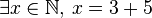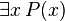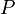# Existence quantifier facts for kids

Kids Encyclopedia Facts

In mathematics and logic, the existence quantifier is a quantifier used to state that a proposition is true for at least one element in the universe of discourse. The existence quantifier is commonly written as$\exists$ (a mirrored E), and is read as "there exists". An example involving an existence quantifier is the statement "some natural number is equal to 3+5", which can be written as$\exists x \in \mathbb{N},\, x = 3+5$.

In general, a statement of the form$\exists x \, P(x)$ is true if there is an x in the universe of discourse satisfying the predicate$P$, and is false otherwise. An existence quantifier is different from a universal quantifier, which is used to state that a proposition is true for all elements in the universe of discourse.

## Related pagesExistence quantifier Facts for Kids. Kiddle Encyclopedia.